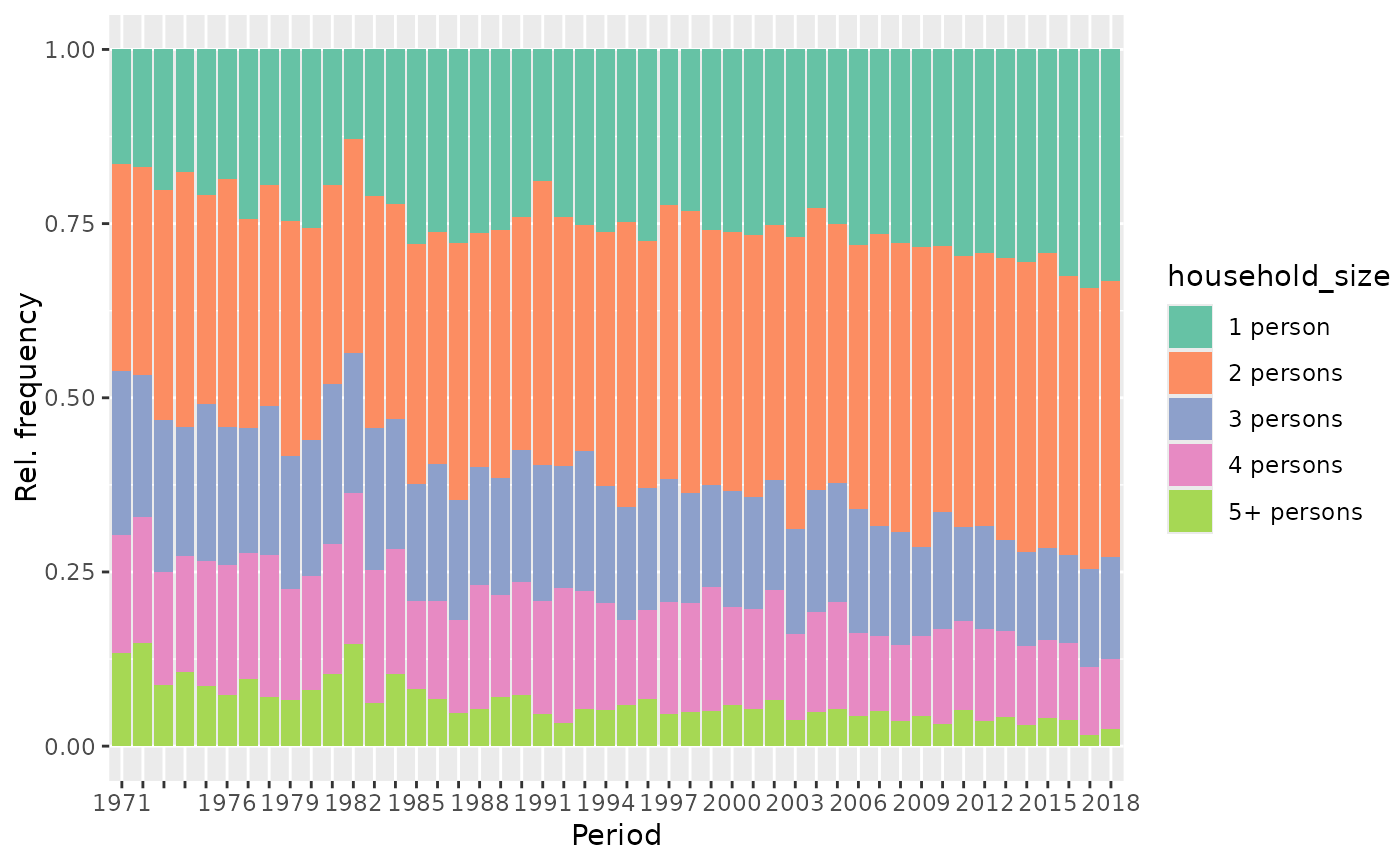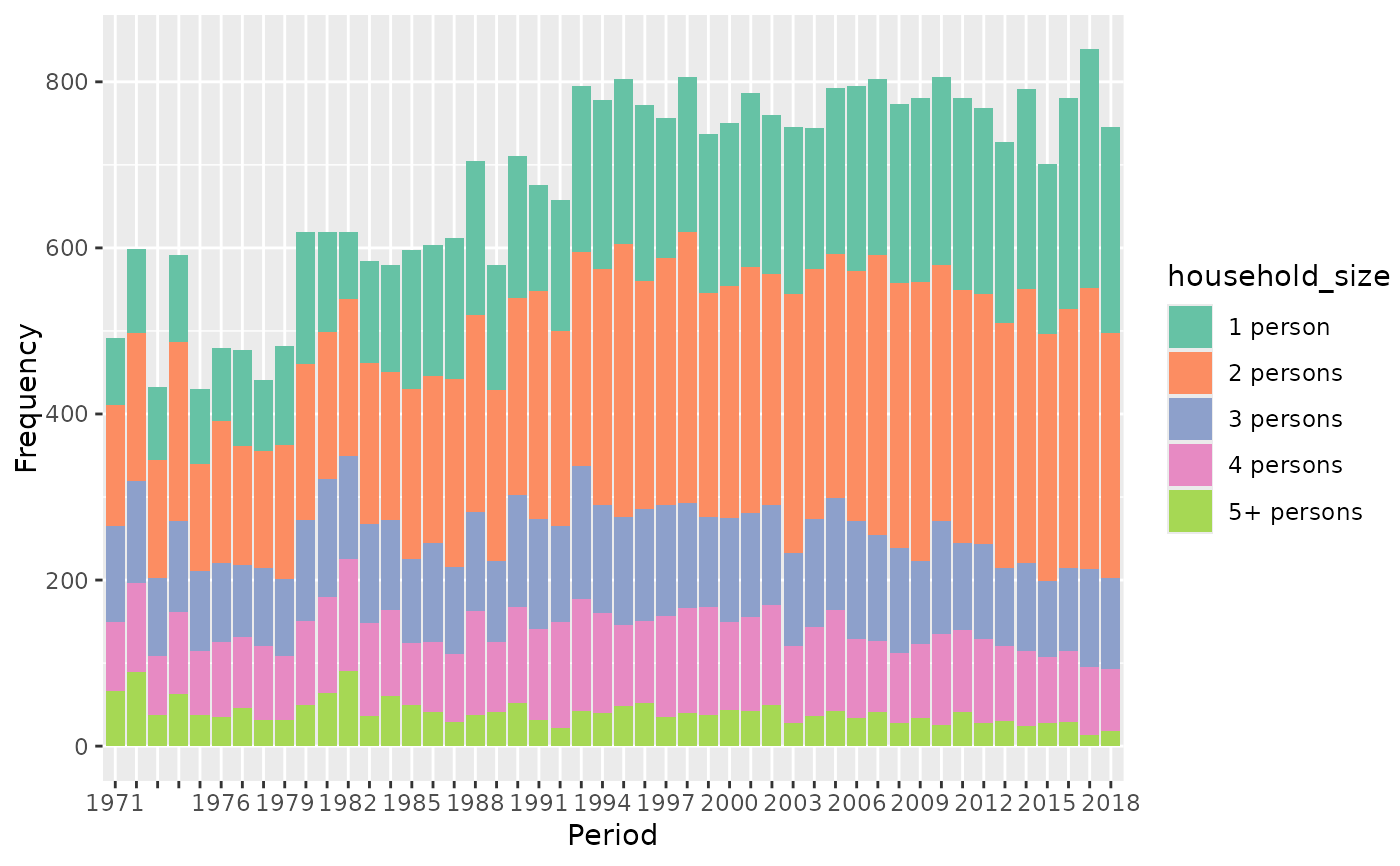Plot the distribution of one variable in the data against age, period or cohort. Creates a bar plot for categorical variables (see argument geomBar_position) and boxplots or a line plot of median values for metric variables (see plot_type).

## Usage

plot_variable(
dat,
y_var,
apc_dimension = "period",
log_scale = FALSE,
plot_type = "boxplot",
geomBar_position = "fill",
legend_title = NULL,
ylab = NULL,
ylim = NULL
)

## Arguments

dat

Dataset containing columns age and period.

y_var

Character name of the variable to plot.

apc_dimension

One of c("age","period","cohort"). Defaults to "period".

log_scale

Indicator if the visualized variable should be log10 transformed. Only used if the variable is numeric. Defaults to FALSE.

plot_type

One of c("boxplot","line","line-points"), specifying if boxplots or a line plot of median values should be drawn for metric variables. "line-points" adds points to the line plot where observations are available.

geomBar_position

Value passed to geom_bar as position argument. Only used if the visualized variable is categorical. Defaults to "fill".

legend_title

Optional character title for the legend which is drawn for categorical variables.

ylab, ylim

Optional arguments for styling the ggplot.

ggplot object

## Author

Alexander Bauer alexander.bauer@stat.uni-muenchen.de

## Examples

library(APCtools)
data(travel)

# plot a metric variable
plot_variable(dat = travel, y_var = "mainTrip_distance",
apc_dimension = "period", log_scale = TRUE)
#> Excluding 9565 missing observations of mainTrip_distance...plot_variable(dat = travel, y_var = "mainTrip_distance",
apc_dimension = "period", log_scale = TRUE, plot_type = "line")
#> Excluding 9565 missing observations of mainTrip_distance...# plot a categorical variable
plot_variable(dat = travel, y_var = "household_size", apc_dimension = "period")
#> Excluding 10 missing observations of household_size...plot_variable(dat = travel, y_var = "household_size", apc_dimension = "period",
geomBar_position = "stack")
#> Excluding 10 missing observations of household_size...